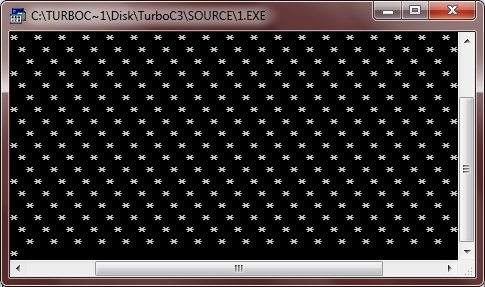# C Loops

In C, loops allows a set of instructions to be repeatedly executed until a certain condition is reached. However, the condition, may be predetermined (as in for loop) or open ended (as in while and do-while loops).

## C Types of Loops

C allows the following three types of loops:

## C Infinite Loop

In C, you can use any loop statement to create an infinite loop.

Here is an example program of infinite loop in C.

```/* C Loops - The infinite loop */

#include<stdio.h>
#include<conio.h>
void main()
{
clrscr();

for(;;)
{
printf("*  ");
}

getch();
}```

As you can see, when the condition expression is absent, then it is assumed to be true.

Here is the sample run of this C program. The printing of stars(*) continue forever.## C Loop Control Statements

There are three types of statements that controls the loop in C, these are:

### More Examples

Here are some more examples that you can go for:

Tools
Calculator